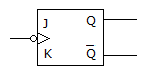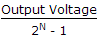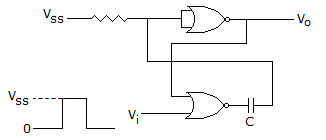# Online Electronics and Communication Engineering Test - Digital Electronics Test 3Loading Test...

Instruction:

• This is a FREE online test. DO NOT pay money to anyone to attend this test.
• Total number of questions : 20.
• Time alloted : 30 minutes.
• Each question carry 1 mark, no negative marks.
• DO NOT refresh the page.
• All the best :-).

1.

DeMorgan's first theorem shows the equivalence of

A.
 OR gate and Exclusive OR gateB.
 NOR gate and Bubbled AND gateC.
 NOR gate and NAND gateD.
 NAND gate and NOT gateExplanation:

First theorem A + B = A . B.

2.

In the given figure, the flip flop isA.
 negative edge triggeredB.
 positive edge triggeredC.
 level triggeredD.
 either (a) or (c)Explanation:

The small circle indicates negative and small triangle indicates edge triggering.

3.

In a 4 input OR gate, the total number of High outputs for the 16 input states are

A.
 16B.
 15C.
 14D.
 13Explanation:

OR gate gives high output when one or more inputs are high.

4.

What will be output for decimal input 82?

A.
 0.82 VB.
 8 VC.
 0.1 VD.
 10 mVExplanation:

Output for decimal input 82/(10000010) = 0.01 x 82 = 0.82 V.

5.

The resolution of 4 bit counting ADC is 0.5 volt, for an Analog input of 6.6 volts. The digital output of ADC will be

A.
 1011B.
 1101C.
 1100D.
 1110Explanation:

Digital output of ADC resolution =.

6.

The circuit in the figure is has two CMOS-NOR gates. This circuit functions as aA.
 flip-flopB.
 schmitt triggerC.
 monostable multivibratorD.
 astable multivibratorExplanation:

It is simple monostable multivibrator.

7.

ALU carries out

A.
 arithmetic operationsB.
 logic operationsC.
 both arithmetic and logic operationsD.
 none of the above8.

The Boolean function A + BC is a reduced form of

A.
 AB + BCB.
 (A + B) . (A + C)C.
 AB + ABCD.
 (A + C) . B9.

Which of the following pairs of octal and binary numbers are not equal?

A.
 111 110112 = 7678B.
 1101101012 = 6658C.
 110102 = 38D.
 10101.112 = 25.6810.

Fastest memory cell

A.
 bubble memoryB.
 core memoryC.
 semiconductor memoryD.
 superconductor memory11.

A mod-2 counter followed by a mod 5 is same as

A.
 mod-7 counterB.
 A decade counterC.
 mod-3 counterD.
 none12.

The values of 25 in octal system is

A.
 40B.
 20C.
 400D.
 20013.

In a four variable K map eight adjacent cells give

A.
 single variable termB.
 two variable termC.
 three variable termD.
 four variable term14.

A Charge Couple Device (CCD) is a

A.
 RAMB.
 sequential accessed memoryC.
 content addressable memoryD.
 read only memory15.

A(A + B) =

A.
 AB.
 A BC.
 ABD.
 AB16.

The 54/74164 chip is an 8-bit serial-input-parallel-output shift register. The clock is 1 MHz. The time needed to shift a 8-bit binary number into the chip is

A.
 1 μsB.
 2 μsC.
 8 μsD.
 16 μs17.

Which device uses Pneumatic fluid?

A.
 LEDB.
 LCDC.
 VF displayD.
 None of these18.

In a clocked NAND latch, race condition occur when

A.
 R and S are high CLK is lowB.
 R and CLK are high and S is lowC.
 R, S, CLK are highD.
 R, S, CLK are low19.

The microprograms provided by a manufacturer to be used on his microprogrammed computer are generally called

A.
 softwareB.
 networkC.
 firmwareD.
 hardware20.

A microcomputer has memory locations from 0000 to FFFF, each storing 1 byte. How many bytes can be the memory store?

A.
 12333B.
 14666C.
 16384D.
 16655